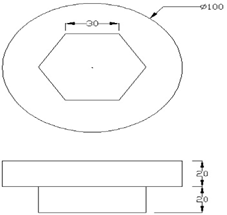### MODEL QUESTIONS FOR FINAL EXAM | BASIC WORK SHOP PRACTICE-II | DIPLOMA

COURSE TITTLE: BASIC WORK SHOP PRACTICE-II

TIME: 3 HOURS                                                                                  MARKS:50

FOR FORGING PRACTICE

1. Prepare the model as per the given sketch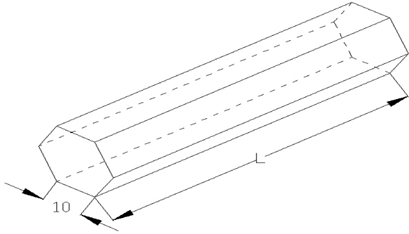2. Prepare the model as per the given sketch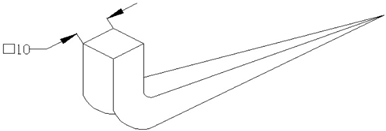1.Prepare the model as per the given sketch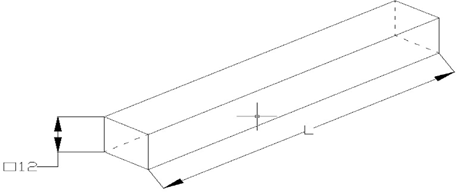FOR SHEET METAL PRACTICE

1.Prepare the model as per the given sketch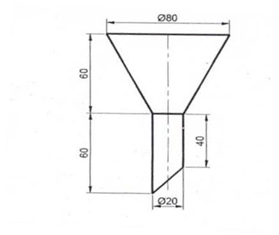1.Prepare the model as per the given sketch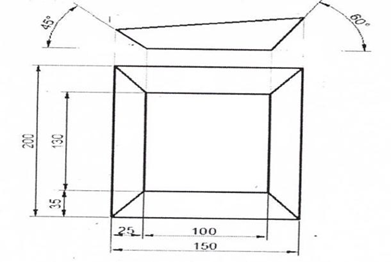FOR FOUNDRYPRACTICE

1. Prepare the model as per the given sketch        2..Prepare the model as per the given sketch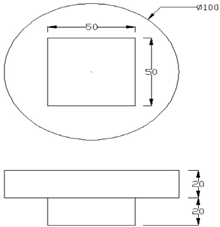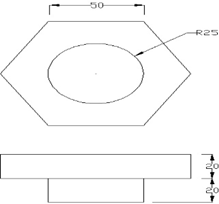3..Prepare the model as per the given sketch